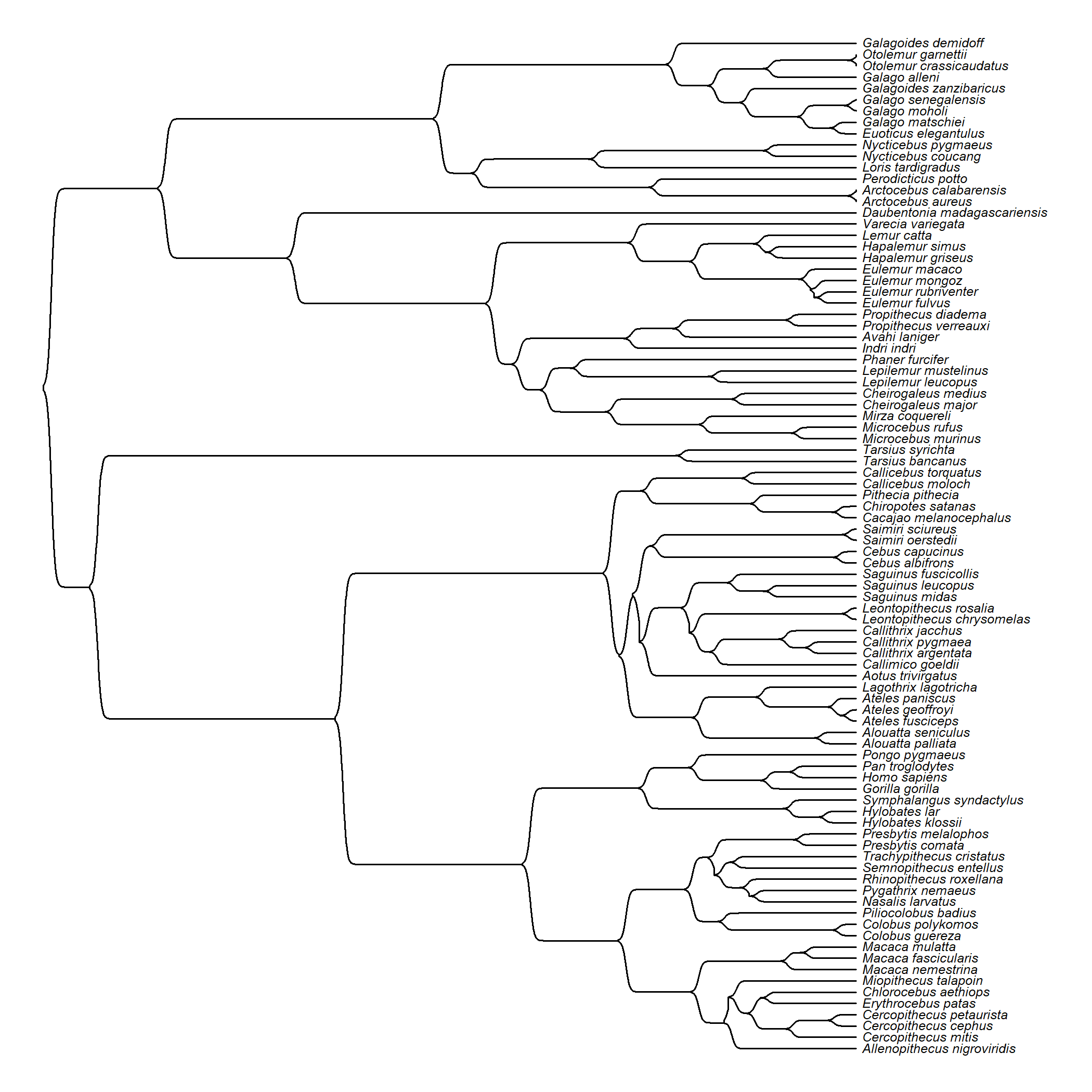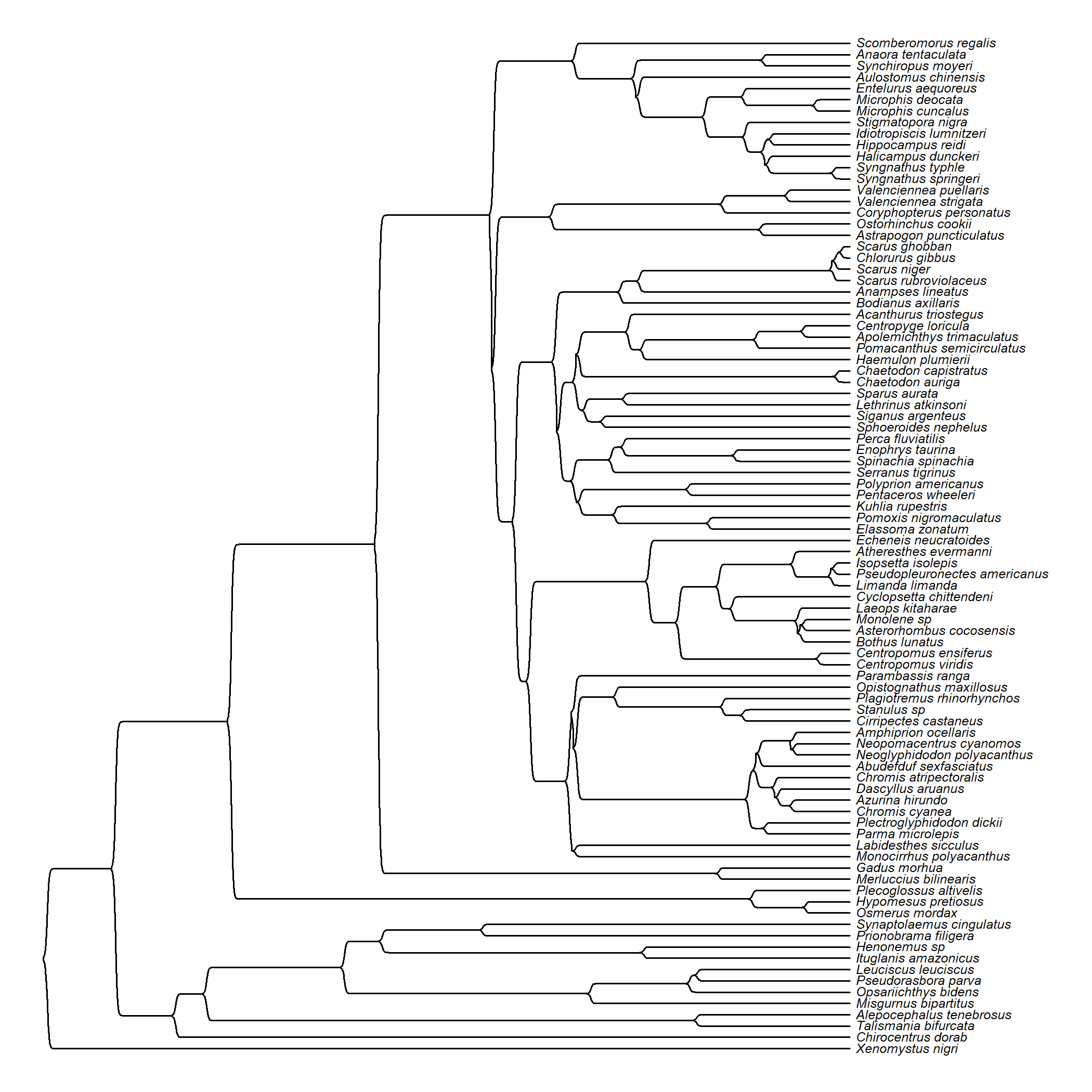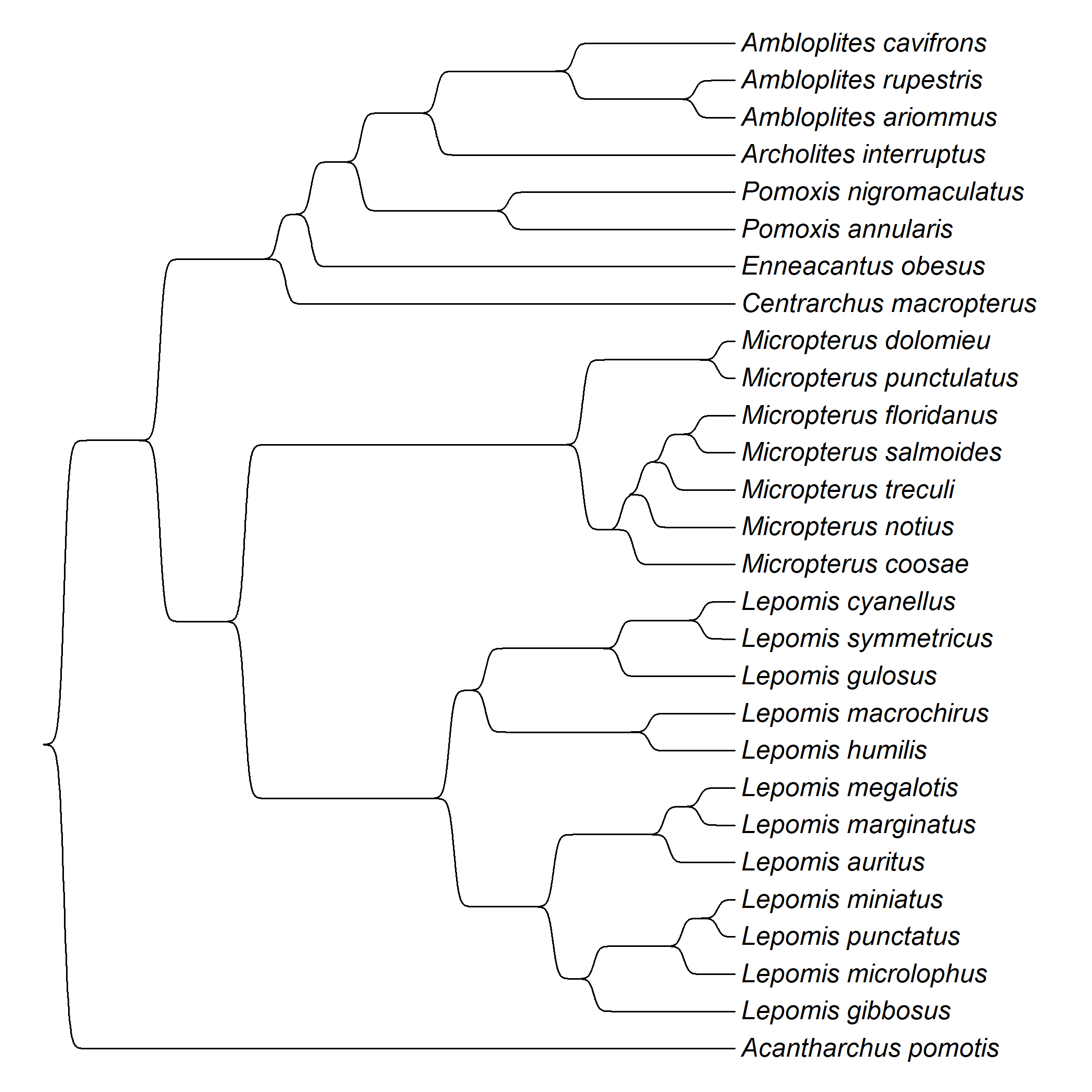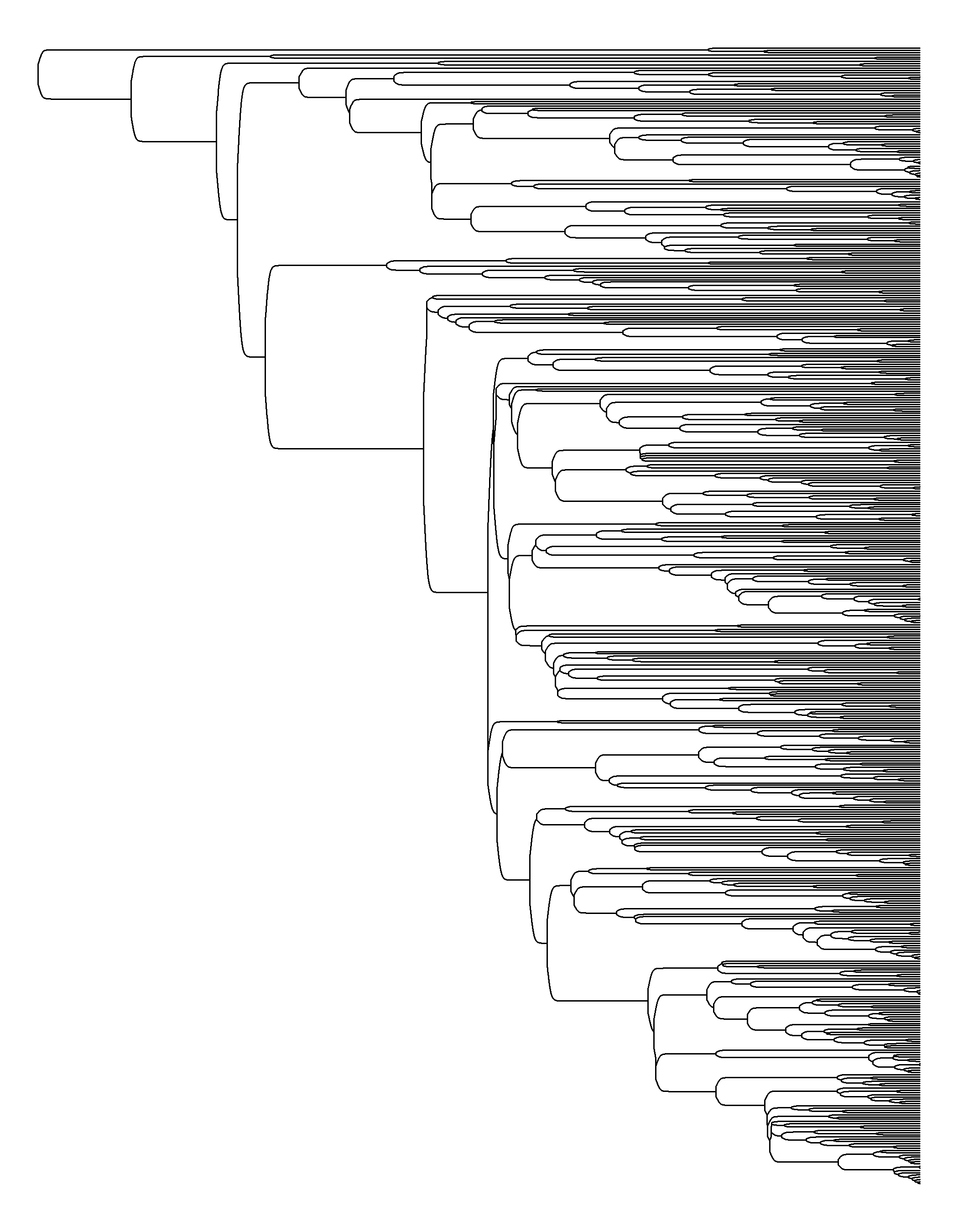## Friday, July 29, 2022

### Plotting the edges of a tree in sigmoid style using the generalized logistic function

On a whim today, I decided to try to write a function to plot a phylogeny with the edges graphed using sigmoid curves. (This is the type of thing that Luke Harmon & I cover in Chapter 13 of our book.)

I decided to use the generalized logistic function from among the wide variety of different sigmoid functions that are available.

I'm not sure I got it exactly right, but here's what I came up with.

``````sigmoidPhylogram<-function(tree,b=5,m1=0.01,m2=0.9,v=1,...){
if(hasArg(use.edge.length))
use.edge.length<-list(...)\$use.edge.length
else use.edge.length<-TRUE
if(!use.edge.length) tree<-compute.brlen(tree)
h<-max(nodeHeights(tree))
args<-list(...)
args\$tree<-tree
args\$color<-"transparent"
do.call(plotTree,args)
pp<-get("last_plot.phylo",envir=.PlotPhyloEnv)
## Yt<-A+(K-A)/(C+exp(-B*(t-M)))^(1/v)
for(i in 1:nrow(tree\$edge)){
A<-pp\$yy[tree\$edge[i,1]]
K<-pp\$yy[tree\$edge[i,2]]
if(i==1) dy<-abs(A-K)
B<-b*Ntip(tree)/h
t<-seq(pp\$xx[tree\$edge[i,1]],pp\$xx[tree\$edge[i,2]],
length.out=1000)
dd<-diff(range(t))
M<-t + if(m1*h>(m2*dd)) m2*dd else m1*h
C<-1
Yt<-A+(K-A)/((C+exp(-B*(t-M)))^(1/v))
t<-c(t,t,t[length(t)])
Yt<-c(A,Yt,K)
lines(t,Yt)
}
}
``````

OK, let's try to use it.

First, using a 90 taxon phylogeny of primates from Kirk & Kay (2004) that also features in our book.

``````library(phytools)
sigmoidPhylogram(primate.tree,ftype="i",fsize=0.5)
``````That's surprisingly not that bad. Here's another example using a phylogeny of bony fish from Benun Sutton & Wilson (2019). The arguments `b` and `m1` control the general shape of the sigmoid function used to plot the edges.

``````bonyfish.tree<-read.tree(file="http://www.phytools.org/Rbook/7/bonyfish.tre")
sigmoidPhylogram(bonyfish.tree,ftype="i",fsize=0.5,b=10,m1=0.005)
``````Here's a much smaller phylogeny of centrarchid fishes from Revell & Collar (2008).

``````sunfish.tree<-read.nexus(file="http://www.phytools.org/Rbook/5/Centrarchidae.nex")
sigmoidPhylogram(sunfish.tree,ftype="i",b=10,m1=0.03,m2=0.5)
``````Lastly, here's a much larger phylogeny of flowering plants from Williams et al. (2014).

``````plant.tree<-read.tree(file="http://www.phytools.org/Rbook/7/pollen-tree.phy")